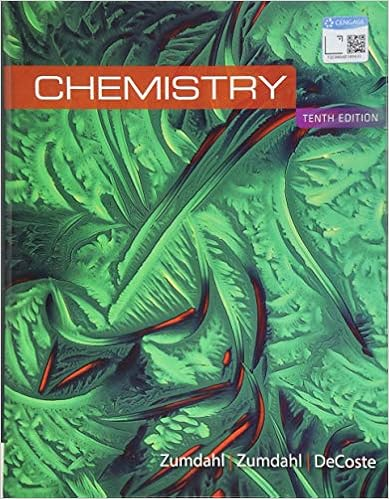# Volume ρ ρ qv dqdv for continuous charge

• Notes
• neda24
• 24
• 100% (2) 2 out of 2 people found this document helpful

This preview shows page 5 - 7 out of 24 pages.

Total physic test

67 .   Which of the following graphs best represents the electric field along the positive x - axis ? Φ E : electric flux ( N m 2 / C ) E: electric field vector ( N/C )
A : area ( m 2 ) Electric Flux through Closed Surface Φ E = ∫ E • d A Gaussian surface The surface of a closed 3 - dimensional shape in space . Net flux over a Gaussian surface is zero if surface contains no charge . Net flux is positive if the Gaussian surface contains + charge . Net flux is negative if the Gaussian surface contains - charge . A Gaussian surface can be any arbitrary shape . Gaussian surfaces are typically chosen for convenience and high symmetry with regard the electric distribution they might enclose .
48 .   A conducting sphere of radius R carries a charge Q . Another conducting sphere has a radius R / 2 , but carries the same charge . The spheres are far apart . The ratio of the electric field near the surface of the smaller sphere to the field near the surface of the larger sphere is most nearly
Questions   51-52 Two concentric , spherical conducting shells have radii r 1 and r 2 and charges Q 1 and Q 2 , as shown above . Let r be the distance from the center of the spheres and consider the region r 1 < r < r 2 . 51 . In this region the electric field is proportional to
41 .   Gauss 's law provides a convenient way to calculate the electric field outside and near each of the following isolated charged conductors EXCEPT a
51 .   In this region the electric field is proportional to
A ) Q 1 /r 2
B ) Q 1 + Q 2 /r 2
C ) Q 1 + Q 2 /r
D ) Q 1 /r 1 + Q 2 /r
E ) Q 1 /r + Q 2 /r 2
38 .   The net electric flux through a closed surface is
40 .   A closed surface , in the shape of a cube of side a , is oriented as shown above in a region where there is a constant electric field of magnitude E parallel to the x - axis . The total electric flux through the cubical surface is
##### We have textbook solutions for you!
The document you are viewing contains questions related to this textbook.The document you are viewing contains questions related to this textbook.
Chapter 8 / Exercise 103
Chemistry
ZumdahlExpert Verified
dE
A
A
E
2
dA
5
##### We have textbook solutions for you!
The document you are viewing contains questions related to this textbook.The document you are viewing contains questions related to this textbook.
Chapter 8 / Exercise 103
Chemistry
ZumdahlExpert Verified
Problem: Gauss’ Law (1998)41. Gauss's law provides a convenient way to calculate the electric field outside and near each of the following isolated charged conductors EXCEPT aExpain your reasoning
Problem: Gauss’ Law (1993)38. The net electric flux through a closed surface is
D) zero if only positive charges are enclosed by the surfaceE) zero if the net charge enclosed by the surface is zeroExplain your reasoningProblem: Gauss’ Law (1993)Questions 51-52Two concentric, spherical conducting shells have radii r1and r2and charges Q1and Q2, as shown above. Let r be the distance from the center of the spheres and consider the region r1< r < r2.51. In this region the electric field is proportional to
2
/r
Show your workProblem: Gauss’ Law (1984)40.A closed surface, in the shape of a cube of side a, is oriented as shown above in a region where there is a constant electric field of magnitude E parallel to the x-axis. The total electric flux through the cubical surface is
•••